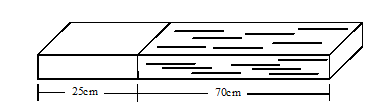The rod given in the figure is made of two different materials. Both have square cross-section of side 3mm. The resistivity of the first material is 4×10-3Ω-m and it is 25cm long while that of the second material is 5×10-3Ω-m and is of length 70cm. The equivalent resistivity of the rod between its ends is __________×10−3Ω−m(roundoff to nearest integer)

# The rod given in the figure is made of two different materials. Both have square cross-section of side 3mm. The resistivity of the first material is $4\mathrm{x}{10}^{-3}\mathrm{\Omega }-\mathrm{m}$ and it is 25cm long while that of the second material is $5\mathrm{x}{10}^{-3}\mathrm{\Omega }-\mathrm{m}$ and is of length 70cm. The equivalent resistivity of the rod between its ends is __________$×{10}^{-3}\mathrm{\Omega }-m$(roundoff to nearest integer)Register to Get Free Mock Test and Study Material

+91

Verify OTP Code (required)

### Solution:

${\mathrm{\rho }}_{\mathrm{eq}}=\frac{{\mathrm{\rho }}_{1}{\mathrm{l}}_{1}+{\mathrm{\rho }}_{2}{\mathrm{l}}_{2}}{{\mathrm{l}}_{1}+{\mathrm{l}}_{2}}$

$⇒{\mathrm{\rho }}_{\mathrm{eq}}=\frac{4\mathrm{x}{10}^{-3}.25+5\mathrm{x}{10}^{-3}.70}{25+70}=4.74\mathrm{x}{10}^{-3}\mathrm{\Omega }-\mathrm{m}\simeq 5\mathrm{x}{10}^{-3}\mathrm{\Omega }-\mathrm{m}$Register to Get Free Mock Test and Study Material

+91

Verify OTP Code (required)